# 大涡模拟脉动速度入口UDF

• 大家好，我是CFD相关专业学生，最近在学习大涡模拟，有不少疑问想要与大家交流、讨论。

首先说下相关背景，我是想要做一个建筑物风场环境的大涡模拟，因为是初次学习，课题组也不能提供多少帮助，所以急需一个讨论学习的空间。

1.我写了一个关于大涡模拟脉动风速入口的udf, 自己也尝试在fluent编译通过，但是初始化流场总是不成功，出现发散结果，不知道是不是UDF的编写有啥问题，希望大家能够一起参谋参谋。

/**************************************************************************************\

UDF基于华南理工大学余远林硕士论文中关于大涡模拟脉动风速入口（窄带叠加法，NSRFG）的相关内容编写。
本人是CFD领域新手，也是第一次编写UDF，若有错误、不妥之处，望不吝指教。

E-mail: hfut_217@hotmail.com

\**************************************************************************************/

#include "udf.h"

#define	OHM	 7.26E-5   /*湍流积分尺度、湍流强度的相关参数，见ratio_rms部分*/
#define	phi	23.167    /*地区纬度*/
#define	u_frac	0.69  /*摩擦速度*/
#define	PI	3.1415926   /*π值*/
#define interval_fre  0.01  /*频率带宽Δf*/
#define NUM 1000  /*功率谱的离散数目*/

DEFINE_PROFILE(inlet_x_velocity, thread, index)
{
real x[ND_ND];
real z, mean = 0;
face_t f;
long int seed = 13579;
real t = CURRENT_TIME;
begin_f_loop(f, thread)
{
F_CENTROID(x, f, thread);
z = x;
mean = 10 * pow(z / 10, 0.15);   /*设定10 m高平均风速为10 m/s*/
real i, fn, sum;
real fluction_v = 0.0;
for (i = 1; i <= NUM; i++)
{
fn = (2 * i - 1) * interval_fre / 2;
sum = p_in(x, 0, fn) * sin(k_in(x, 0, fn) * x / l_in(x, 0, fn)) + 2 * PI * fn * t + uniform(0.0, 2 * PI, &seed);
fluction_v = fluction_v + sum;
}
F_PROFILE(f, thread, index) = fluction_v + mean;
}
end_f_loop(f, thread)
}

DEFINE_PROFILE(inlet_y_velocity, thread, index)
{
real x[ND_ND];
real z = 0;
face_t f;
long int seed = 13579;
real t = CURRENT_TIME;
begin_f_loop(f, thread)
{
F_CENTROID(x, f, thread);
real i, fn, sum;
real fluction_v = 0.0;
for (i = 1; i <= NUM; i ++)
{
fn = (2 * i - 1) * interval_fre / 2;
sum = p_in(x, 1, fn) * sin(k_in(x, 1, fn) * x / l_in(x, 1, fn)) + 2 * PI * fn * t + uniform(0.0, 2 * PI, &seed);
fluction_v = fluction_v + sum;
}
F_PROFILE(f, thread, index) = fluction_v + 0.0;
}
end_f_loop(f, thread)
}

DEFINE_PROFILE(inlet_z_velocity, thread, index)
{
real x[ND_ND];
real z = 0;
face_t f;
long int seed = 13579;
real t = CURRENT_TIME;
begin_f_loop(f, thread)
{
F_CENTROID(x, f, thread);
real i, fn, sum;
real fluction_v = 0.0;
for (i = 1; i <= NUM; i++)
{
fn = (2 * i - 1) * interval_fre / 2;
sum = p_in(x, 2, fn) * sin(k_in(x, 2, fn) * x / l_in(x, 2, fn)) + 2 * PI * fn * t + uniform(0.0, 2 * PI, &seed);
fluction_v = fluction_v + sum;
}
F_PROFILE(f, thread, index) = fluction_v + 0.0;
}
end_f_loop(f, thread)
}

real uniform(real a, real b, long int *seed)  /*产生符合均匀分布U（a,b）的随机数*/
{
real t = 0;
*seed = 2045 * (*seed) + 1;
*seed = (*seed) % 1048576;
t = (*seed) / 1048576.0;
t = a * (b - a) * t;
return t;
}

real p_in(real vec1[], int dir, real fn) /*论文中脉动风速表达式右边第一个参数 p */
{
real s = 0.0;
s = sqrt(2 * spectrum(vec1, dir, fn) * interval_fre);
return s;
}

real l_in(real vec1[], int dir, real fn) /*论文中脉动风速表达式右边第三个参数的相关参数*/
{
real c = { 8.0, 10.0, 15.0 };
real gama = { 3.2, 1.6, 1.4 };
real s = 0;
s = mean_v(vec1) / (fn * c[dir] * gama[dir]);
return s;
}

real k_in(real vec1[], int dir, real fn)  /*论文中脉动风速表达式右边第二个参数 K */
{
real NV_VEC(q_in) = { 0.0 };
real s, pl0, pl1, pl2;
pl0 = p_in(vec1, 0, fn) / l_in(vec1, 0, fn);
pl1 = p_in(vec1, 1, fn) / l_in(vec1, 1, fn);
pl2 = p_in(vec1, 2, fn) / l_in(vec1, 2, fn);
q_in = pow(pl0, 2);
q_in = pow(pl1, 2);
q_in = pow(pl2, 2);
real A = sqrt(pow(q_in + q_in , 2) + q_in * q_in + q_in * q_in);
real B = sqrt(q_in * q_in);
long int seed = 13579;
if (dir == 0)
{
s = -(q_in * q_in) * sin(uniform(0.0, 2 * PI, &seed)) / A;
}
else if (dir == 1)
{
s = sqrt(q_in) * sqrt(q_in) *sin(uniform(0.0, 2 * PI, &seed)) / A + sqrt(q_in) *cos(uniform(0.0, 2 * PI, &seed)) / B;
}
else
{
s = sqrt(q_in) * sqrt(q_in) *sin(uniform(0.0, 2 * PI, &seed)) / A - sqrt(q_in) *cos(uniform(0.0, 2 * PI, &seed)) / B;
}
return s;
}

real spectrum(real vec1[], int dir, real fn) /*风速功率谱*/
{
real spec_mole, spec_deno, spec, s;
spec_deno = pow((1 + 70.8 * pow(fn * int_length(vec1, dir) / mean_v(vec1), 2)), 5 / 6);
spec_mole = 4 * pow(tur_intensity(vec1, dir) * mean_v(vec1), 2) * tur_intensity(vec1, dir) * mean_v(vec1);
if (dir == 0)
{
spec = 1;
}
else
{
spec = 1 + 188.4 * pow(2 * fn * (int_length(vec1, dir) * mean_v(vec1)), 2);
}
s = spec_mole * spec / spec_deno;
return s;
}

real mean_v(real vec1[]) /*平均风速*/
{
real m_v, z;
z = vec1;
m_v = 10 * pow(z / 10, 0.15);  /*设定10 m高平均风速为10 m/s*/
return m_v;
}

real tur_intensity(real vec1[], int dir)  /*湍流强度*/
{
real s, z;
z = vec1;
s = 0.14 * pow(z / 10, -0.15) * ratio_rms(vec1, dir);
return s;
}

real int_length(real vec1[], int dir)   /*湍流积分长度*/
{
real length, z;
z = vec1;
if (dir == 0)
{
length = 300 * pow(z / 300, 0.46 + 0.074 * log(0.7));
}
else
{
length = 0.5 * pow(ratio_rms(vec1, dir), 3) * 300 * pow(z / 300, 0.46 + 0.074 * log(0.7));
}
return length;
}

real ratio_rms(real vec1[], int dir)  /*其它风向脉动风速的均方根值与顺风向脉动风速的均方根值 的比值*/
{
real val, ratio, z;
z = vec1;
val = 12 * z * OHM * sin(phi * PI / 180) / u_frac;
if (dir == 0)
{
ratio = 1;
}
else if (dir == 1)
{
ratio = 1 - 0.22 * pow(cos(PI * val / 2), 4);
}
else
{
ratio = 1 - 0.45 * pow(cos(PI * val / 2), 4);
}
return ratio;
}


2.大涡模拟的风速入口的设置疑问。1.在FLUENT中，如果我选择按照余远林硕士论文中NSRFG方法生成脉动风速入口，那是不是在Fluctualing Velocity Algorithm 中选择 No Perturbations,如下图所示。又或者是选择其它选项，如Spectral Synthesizer中再选择Intensity and Length Scale（编写相关表达式的udf）。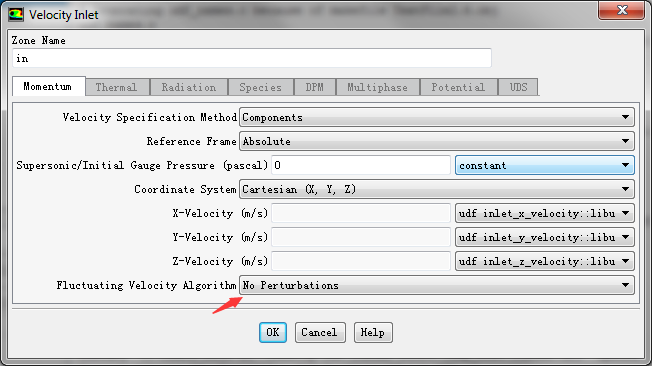2.我看余远林硕士论文中脉动风速入口是先利用MATLAB生成脉动风速数据，然后在FLUNT中利用UDF加载到风场入口边界，而我直接在FLUNT中生成脉动风速数据并直接加载到入口边界，我的这种操作是否有问题，这会不会与我初始化流场不成功有关。大涡模拟的脉动风速到底该如何加载，怎么才能正确地做一次大涡模拟的数值试验。

余远林硕士论文中脉动风速入口实现流程图如下：PS：也许我的问题显得有些弱智，但我真的对于大涡模拟是好多好多不理解，也碰了不少壁，身边也没有人可以交流学习，只能自己闭门造车，心态有点急躁了，希望论坛中大哥大姐多多赐教，多谢多谢多谢。

• @低碳生活 补充初始化流场信息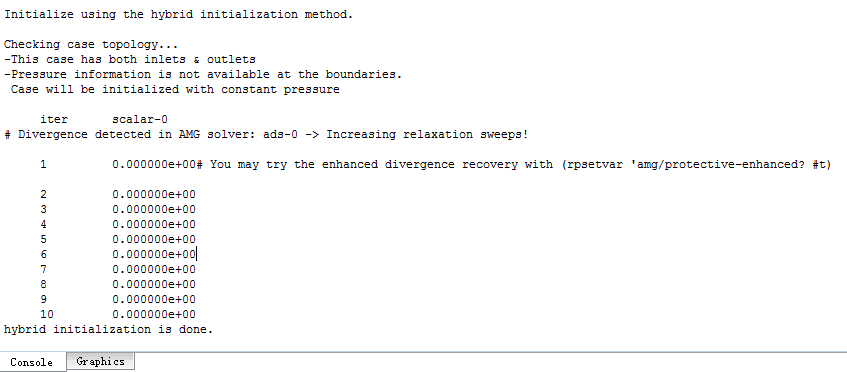• 想问个题外话，为什么Fluent自带的扰动方法不适用你的case呀？

关于代码，不知道他具体表达式是怎样的，所以没法看细节。如果你用他论文里的方法，先在别的地方（MATLAB之类的）生成扰动速度，再用udf读进Fluent应该也是可以的呀。另外，初始化如果不用hybrid呢，就用普通的那种。

• @cccrrryyy 使用Fluent 自带的扰动方法是不是指入口边界使用平均速度入口，脉动速度在Fluctualing Velocity Algorithm 选择相应方法生成。 因为我想要学习他的脉动风速入口的生成方法，现在脉动风速生成方法好多都是选择RFG方法生成，老师要我们紧跟潮流，而且还有后续关于多相流的研究要做。
代码关于公式、表达式的部分应该没啥问题，我担心是一些关于Fluent的宏使用的对不对，还有随机数的部分不知道有没有问题，以及关于UDF中随时间变化的部分。
目前的情况是FLUENT编译通过了，但初始化流场耗时时间很长还且出错，使用Standard Initialization初始化没有任何提示信息，但开始计算也会出现发散，错误信息如下。
（使用Hybird Initialization是因为计算域有比较明确的入口和出口）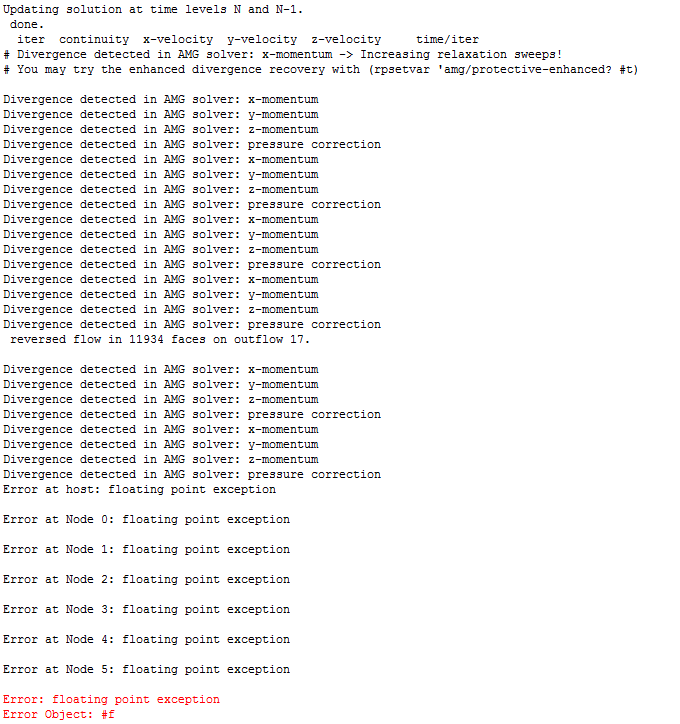• 为方便向大家学习、讨论。我把一些模型、边界等相关信息贴上来。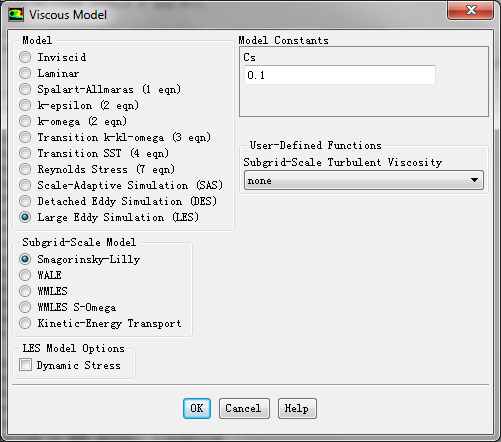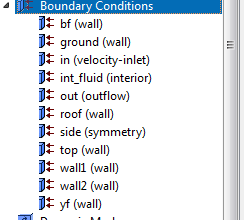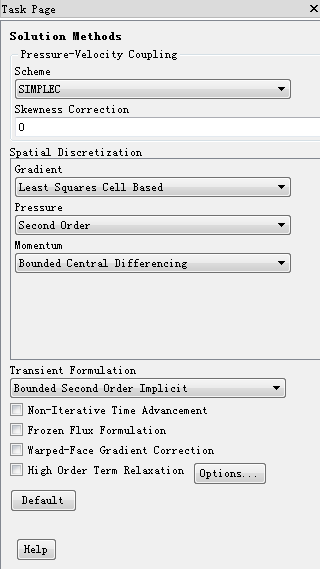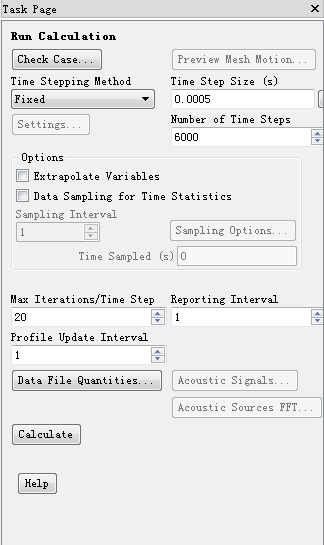• @低碳生活 按你描述的应该没啥问题啊，至少Fluent用UDF给入口速度是很常规的操作，这里不应该有问题。会不会是随机数这一块？Fluent有自带随机数的，需要你在UDF开头 #include "random.h" ，查了一下好像是uniform_random()这个函数，可以产生0到1之间的平均分布的随机数。应该还会有其他的函数吧。总感觉随机数这种最好是有现成的就用现成的，自己写容易出问题。按你说的原文中是用MATLAB去做，可能也是因为MATLAB产生随机数很方便，一个rand()函数就做完了，不需要你给seed。

另外就是代码细节方面了，比如整数尽量写成1.0啊2.0啊之类的，特别是涉及到除法的。

Fluent自带的比如vortex method属于合成类型的方法，我不太清楚和你说的RFG本质上有没有区别，印象中一直觉得合成方法比较真实和高效的（也有可能我落伍了）。看看有没有真正搞湍流入口的人来给你解答啦~

• 其实我觉得展示UDF 特别是数值型的 有一个matlab的预测验证图会比较直观

• @cccrrryyy 谢谢意见，明天有个师兄要回来了，到时候问问他，有消息我会在帖子里更新的。
matlab不太会用，回头瞅瞅

• @l-j刘侃 对matlab不怎么熟悉

• uniform_random()

方便告诉我下fluent帮助文件中关于随机数的部分吗？我好像没找到

• 我也没找到，我是随便搜了一下看到有这么个功能的。好像有两种方式，一种就是用C语言自带的随机数功能，我在这里看到有人提到过；还有一种是我之前说的用Fluent的random头文件，这个我是在这个链接看到的。

Fluent的这些头文件是可以找到的，random.h的路径是 安装目录:\ANSYS Inc\v170（版本号）\fluent\fluent17.0.0\src\util，可以看到有个uniform_random（）函数，应该就是它了。我没用过所以不确定哈。

• udf出错基本上就是除以了0值，或者出现了极大值与极小值，也有可能是调用梯度梯度值不存在而报错，基本上你编写的方程没问题的话，就按照这思路找吧，一点一点的message，